Get instant live expert help with Excel or Google Sheets“My Excelchat expert helped me in less than 20 minutes, saving me what would have been 5 hours of work!”

Post your problem and you’ll get expert help in seconds.

Your message must be at least 40 characters
Our professional experts are available now. Your privacy is guaranteed.

How to Convert Decimals to Fraction – Excelchat

We can convert decimal to fraction and fraction to decimal by using the Number ribbon. This tutorial will guide all levels of Excel users on how to insert fraction in Excel.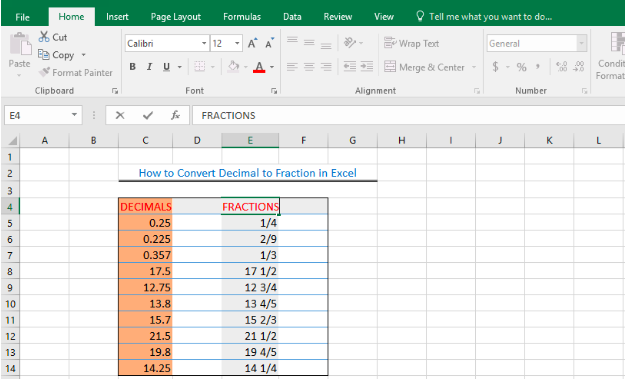Figure 1: How to Convert Decimal to Fraction

How to Put a Fraction in Excel

• To convert a number in decimal to fraction, we will highlight the cell containing the number. We will highlight Cell E5.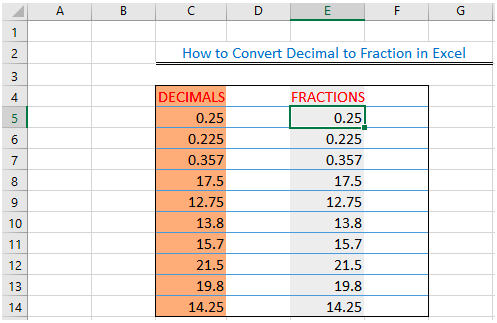Figure 2: Decimal table

• We will click the Home tab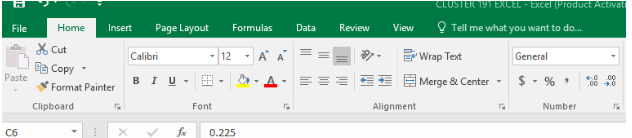Figure 3: Click Home

• We will go to the Number Group
• We will go to General; we will click on the dropdown to select Fraction from the options
• We will click OK to obtain a fraction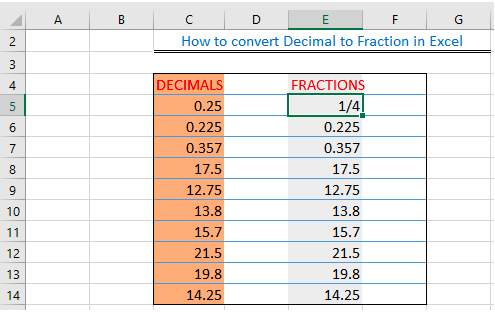Figure 4: Create a single fraction in the cell

Add Fractions in Excel

• To add the fractions to the remaining cells, we will select the cells with the decimals and follow the previous procedure of clicking General and select Fractions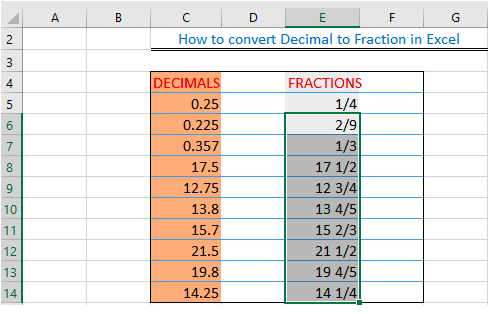Figure 5: Inserted excel display fractions without reducing

How to Convert Fraction to Decimal

• To convert Fractions to Decimals, we will select the cells with the fractions and follow the procedure of clicking Fraction and select Number or General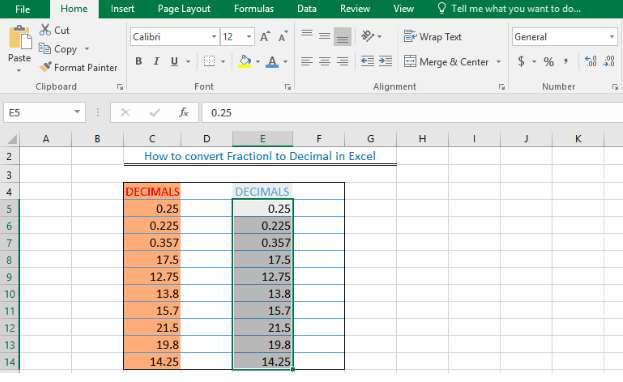Figure 6: How to convert fraction to decimal in excel

How to Display Fraction without Reducing

• To display fraction without reducing, we will select all the Cells (E5:E14) we want to format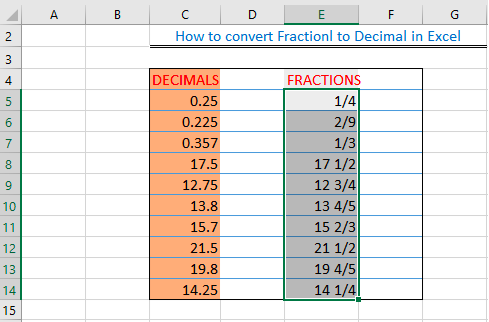Figure 7: Excel Fraction Format

• We will right-click and select Format Cells.
• In the category list, we will choose Custom, the Type box should contain the characters #??/??”
• We will change the contents of the Type box to #??/28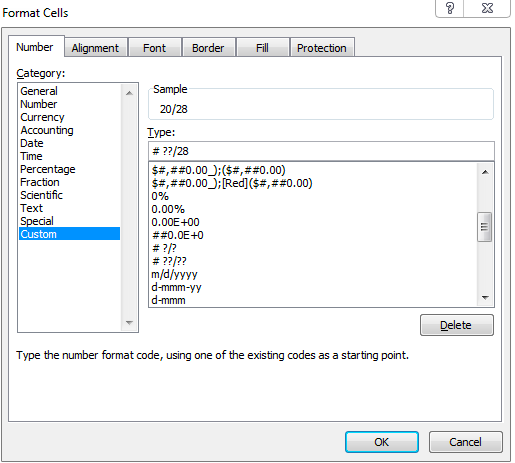Figure 8: Format Cells Dialog box

• We will click the OK button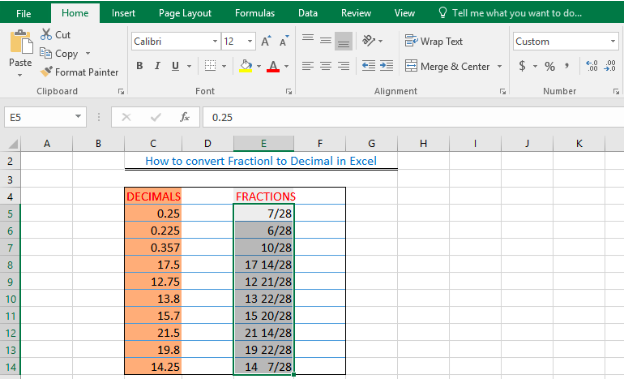Figure 9: How to display fraction without reducing

Instant Connection to an Expert through our Excelchat Service

Most of the time, the problem you will need to solve will be more complex than a simple application of a formula or function. If you want to save hours of research and frustration, try our live Excelchat service! Our Excel Experts are available 24/7 to answer any Excel question you may have. We guarantee a connection within 30 seconds and a customized solution within 20 minutes.